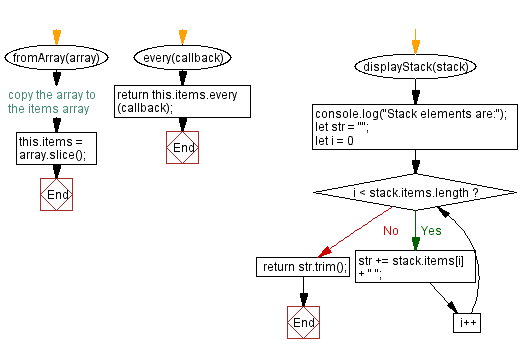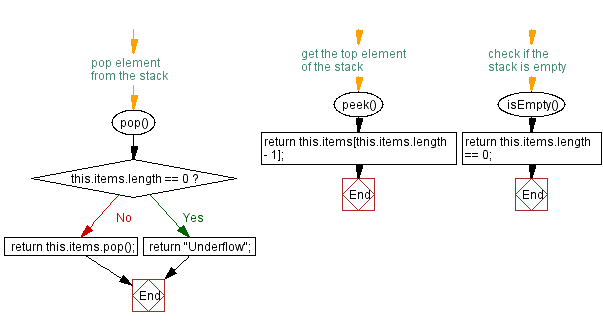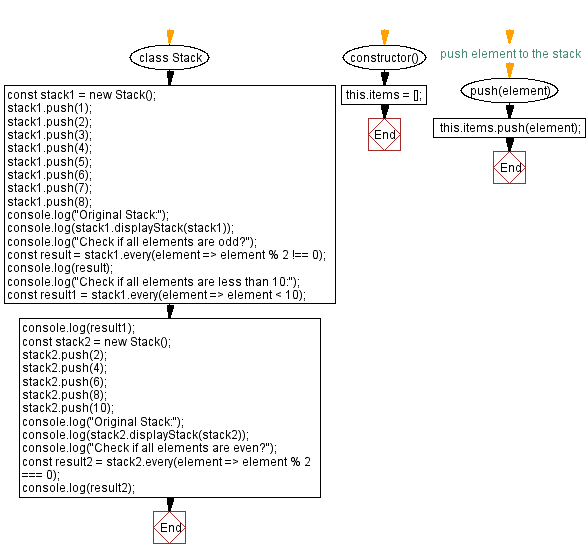# JavaScript Exercises: Verify all stack elements satisfy a condition

## JavaScript Stack: Exercise-33 with Solution

Write a JavaScript program that implements a stack and checks if all elements of the stack satisfy a condition.

Sample Solution:

JavaScript Code:

``````class Stack {
constructor() {
this.items = [];
}

// push element to the stack
push(element) {
this.items.push(element);
}

// pop element from the stack
pop() {
if (this.items.length == 0) {
return "Underflow";
}
return this.items.pop();
}

// get the top element of the stack
peek() {
return this.items[this.items.length - 1];
}

// check if the stack is empty
isEmpty() {
return this.items.length == 0;
}

// get the size of the stack
size() {
return this.items.length;
}
fromArray(array) {
this.items = array.slice(); // copy the array to the items array
}
every(callback) {
return this.items.every(callback);
}
displayStack(stack) {
console.log("Stack elements are:");
let str = "";
for (let i = 0; i < stack.items.length; i++)
str += stack.items[i] + " ";
return str.trim();
}
}

const stack1 = new Stack();
stack1.push(1);
stack1.push(2);
stack1.push(3);
stack1.push(4);
stack1.push(5);
stack1.push(6);
stack1.push(7);
stack1.push(8);
console.log("Original Stack:");
console.log(stack1.displayStack(stack1));
console.log("Check if all elements are odd?");
const result = stack1.every(element => element % 2 !== 0);
console.log(result);
console.log("Check if all elements are less than 10:");
const result1 = stack1.every(element => element < 10);
console.log(result1);
const stack2 = new Stack();
stack2.push(2);
stack2.push(4);
stack2.push(6);
stack2.push(8);
stack2.push(10);
console.log("Original Stack:");
console.log(stack2.displayStack(stack2));
console.log("Check if all elements are even?");
const result2 = stack2.every(element => element % 2 === 0);
console.log(result2);
```
```

Sample Output:

```Original Stack:
Stack elements are:
1 2 3 4 5 6 7 8
Check if all elements are odd?
false
Check if all elements are less than 10:
true
Original Stack:
Stack elements are:
2 4 6 8 10
Check if all elements are even?
true
```

Flowchart:Live Demo:

See the Pen javascript-stack-exercise-33 by w3resource (@w3resource) on CodePen.

Improve this sample solution and post your code through Disqus

Stack Previous: Extract a portion from the said stack.
Stack Exercises Next: Verify at least one element satisfy a condition.

What is the difficulty level of this exercise?

Test your Programming skills with w3resource's quiz.

﻿

## JavaScript: Tips of the Day

Creates an array of elements, ungrouping the elements in an array produced by zip and applying the provided function

Example:

```const tips_unzip = (arr, fn) =>
arr
.reduce(
(acc, val) => (val.forEach((v, i) => acc[i].push(v)), acc),
Array.from({
length: Math.max(...arr.map(x => x.length))
}).map(x => [])
)
.map(val => fn(...val));

console.log(tips_unzip([[2, 15, 200], [3, 25, 300]], (...args) => args.reduce((acc, v) => acc + v, 0)));
```

Output:

```[5, 40, 500]
```